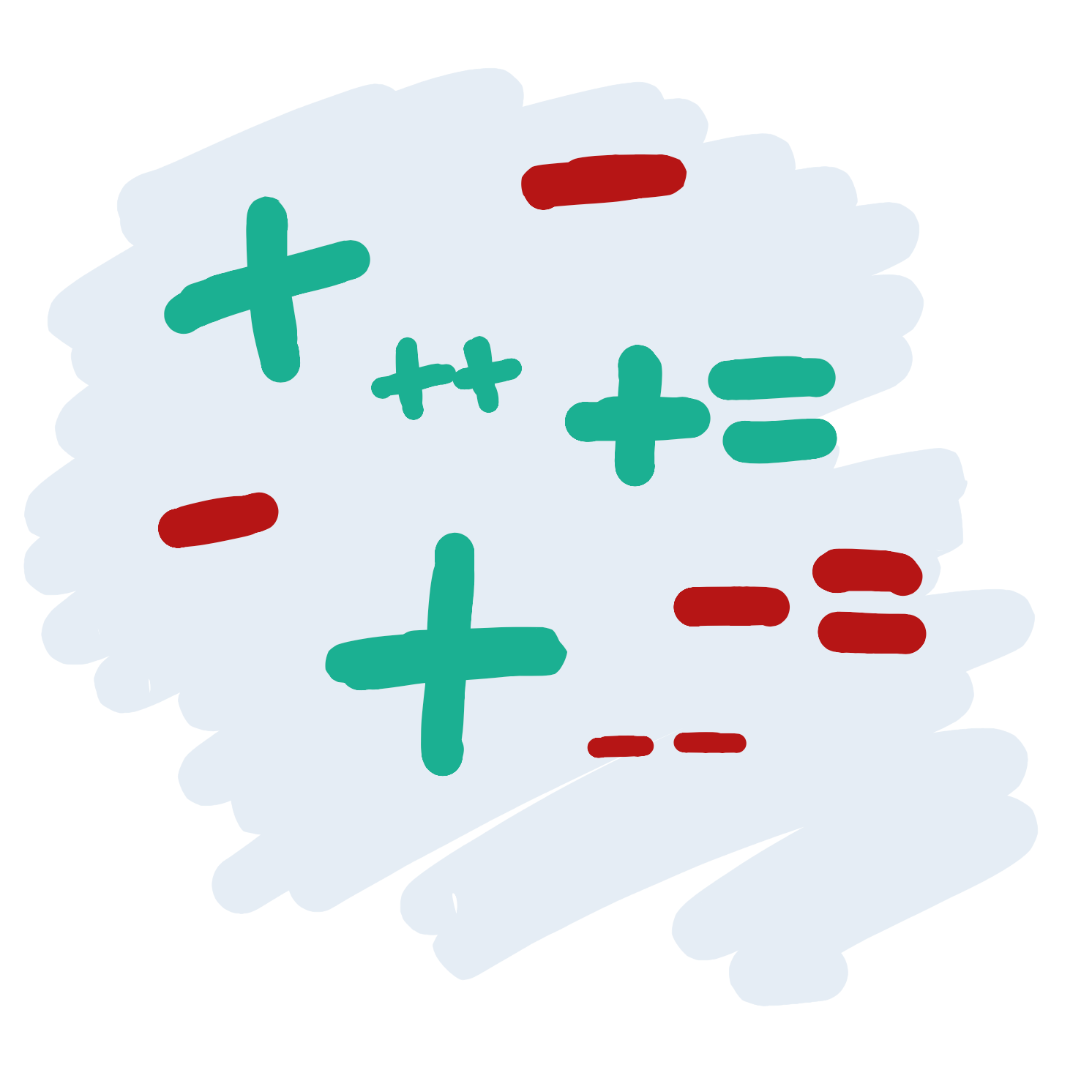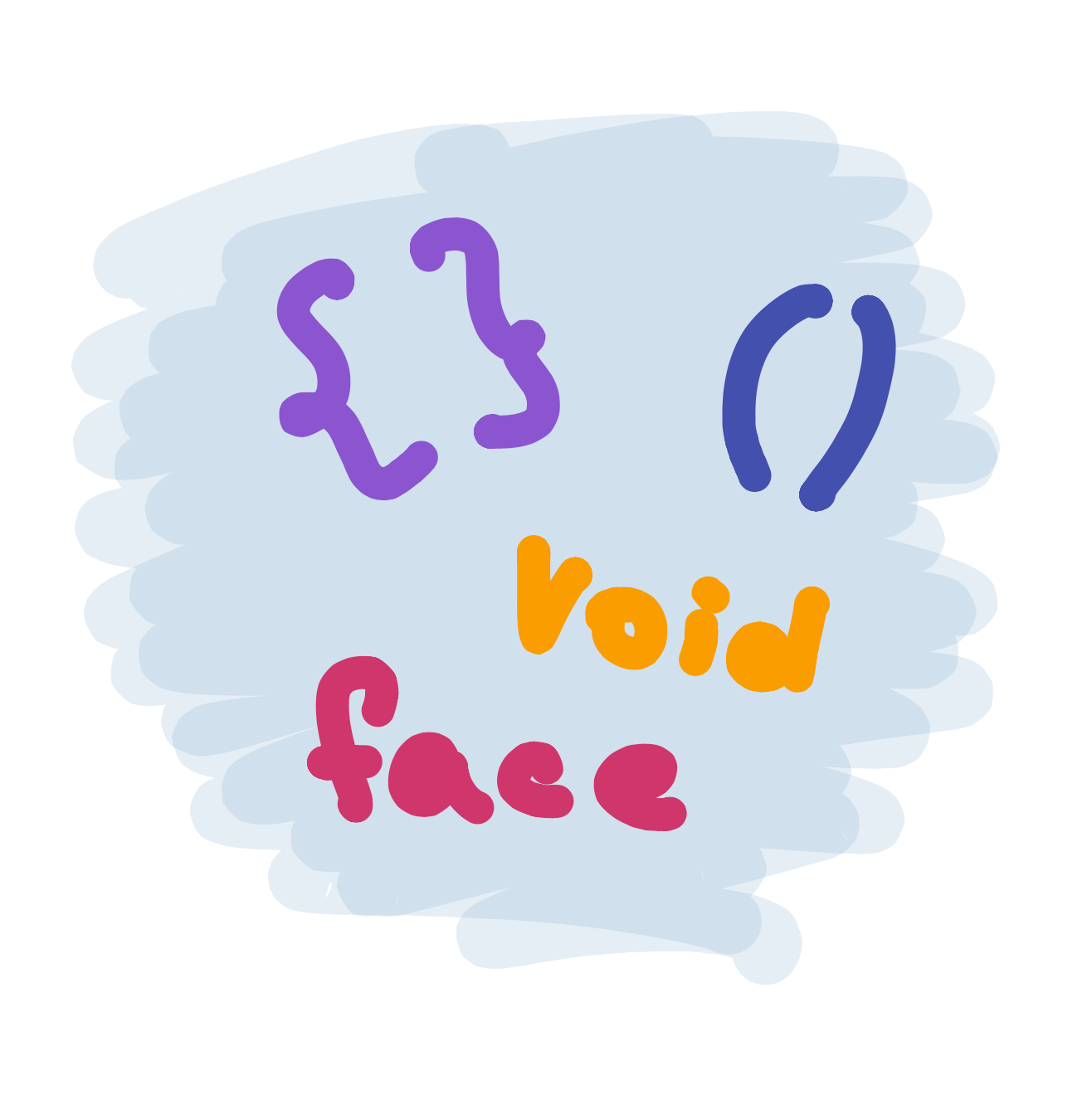# Modifying VariablesSo far, we’ve learned how to draw a face with the `ellipse` and the `rect` functions, and we’ve learned how to change the size of the eyes and how far from the left of the screen it should be with variables. The code we wrote to do this looks a little like this:

##### Code:
```int eyeSize = 20;
int left = 20;
ellipse(left + 5,30,eyeSize,eyeSize);
ellipse(left + 50,30,eyeSize,eyeSize);
rect(left,60,60,20);
```
##### Preview:

What a good looking face, but it might be a little lonely. Let’s add another face so our character has a friend.

Luckily for us, this is pretty easy! We can just copy and paste the three lines of code we use to draw our face and it will draw it a second time. This will draw a second face over the first one, so we will need to change the x attributes for each shape so that they do not overlap

##### Code:
```size(200,100);

int eyeSize = 20;
int left = 20;
ellipse(left + 5,30,eyeSize,eyeSize);
ellipse(left + 50,30,eyeSize,eyeSize);
rect(left,60,60,20);

ellipse(left + 105,30,eyeSize,eyeSize);
ellipse(left + 150,30,eyeSize,eyeSize);
rect(left + 100,60,60,20);
```
##### Preview:

Now that we are using variables, there’s actually an easier way to do this. Rather than changing each x attribute, we can change the value of our `left` variable after we draw the first face. To do this, we just need give the variable a new number, like this:

`left = 120;`

So now, our code might look like this:

##### Code:
```size(200,100);

int eyeSize = 20;
int left = 20;
ellipse(left + 5,30,eyeSize,eyeSize);
ellipse(left + 50,30,eyeSize,eyeSize);
rect(left,60,60,20);

left = 120;

ellipse(left + 5,30,eyeSize,eyeSize);
ellipse(left + 50,30,eyeSize,eyeSize);
rect(left,60,60,20);

```
##### Preview:

This is a better way to draw two faces, since we don’t need to change each line when we copy and paste the code for the second face.

There’s another way we can change the value of a variable. Let’s say we don’t know exactly where we want our second face to be - we just want it to be 50 points left of the first face. We can instead set the variable to 50 more than it used to be:

`left = left + 100`

This way, if we draw three faces, we can copy the four lines again (I’ve made the program a little bigger so we can fit our three faces):

##### Code:
```size(300,100);

int eyeSize = 20;
int left = 20;
ellipse(left + 5,30,eyeSize,eyeSize);
ellipse(left + 50,30,eyeSize,eyeSize);
rect(left,60,60,20);

left = left + 100;
ellipse(left + 5,30,eyeSize,eyeSize);
ellipse(left + 50,30,eyeSize,eyeSize);
rect(left,60,60,20);

left = left + 100;
ellipse(left + 5,30,eyeSize,eyeSize);
ellipse(left + 50,30,eyeSize,eyeSize);
rect(left,60,60,20);

```
##### Preview:

We can also set our variables to be the values of other variables. So we could make the width of our eyes bigger as we move from the left of the screen by typing this:

`eyeSize = left / 4;`

##### Code:
```size(300,100);

int left = 20;
int eyeSize = left / 4;
ellipse(left + 5,30,eyeSize,eyeSize);
ellipse(left + 50,30,eyeSize,eyeSize);
rect(left,60,60,20);

left = left + 100;
eyeSize = left / 4;
ellipse(left + 5,30,eyeSize,eyeSize);
ellipse(left + 50,30,eyeSize,eyeSize);
rect(left,60,60,20);

left = left + 100;
eyeSize = left / 4;
ellipse(left + 5,30,eyeSize,eyeSize);
ellipse(left + 50,30,eyeSize,eyeSize);
rect(left,60,60,20);

```
##### Preview:
Tip

We haven’t seen something like ’/ 4’ part before. All this means is that the left variable will be divided by 4. We are only doing this because otherwise the eyes would be way too big (try writing this code without the division!)

## Simplifying our code

If we are increasing or decreasing the value of a variable, typing this can be a little annoying:

`left = left + 100;`

Instead, we can type this:

`left += 100;`

And if we were subtracting a number, like this:

`left = left - 20;`

We could type this…

`left -= 20;`

There’s an even shorter version if we are just adding one to the variable. Instead of this:

`left += 1;`

We can just type this:

`left++;`

And if we were subtracting one, instead of this:

`left -= 1;`

We could type this:

`left-—;`

As you can see, programmers can be lazy sometimes. This can actually be a good thing! The less code we write, the less things can go wrong.

Next lesson Functions